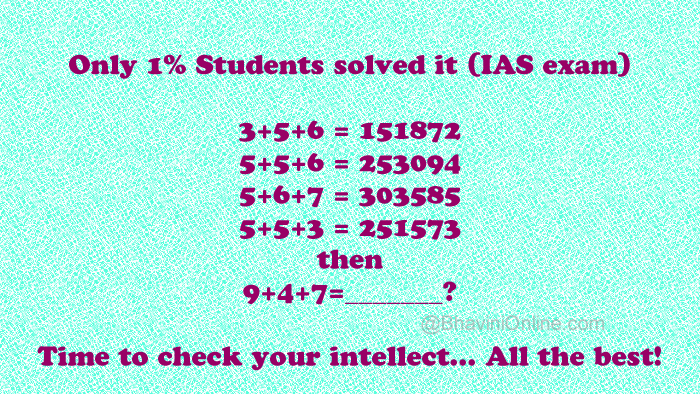# Whatsapp Mathematical Puzzle: If 3+5+6=151872, 9+4+7=?

I came across this tough maths puzzle on Whatsapp today.

Only 1% Students solved it (IAS exam)

3+5+6=151872

5+5+6=253094

5+6+7=303585

5+5+3=251573

then 9+4+7=_______?

Time to check your intellect… All the best!So were you able to solve the riddle? Leave your answers in the comment section below.